### Home > PC > Chapter 2 > Lesson 2.3.5 > Problem2-130

2-130.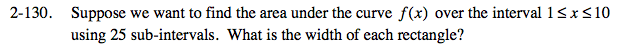$\text{width }=\frac{\text{length of interval}}{\text{No. of rectangles}}$

$\text{width }=\frac{9}{25\text{ rectangles}}=.36$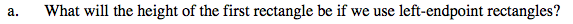x0 = 1 → height of rectangle = f(1)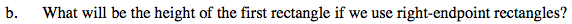Height of x1.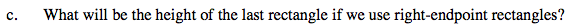For right-endpoint, we start at x1 and go to the end. So you want the height at that xend.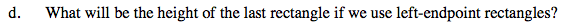For left-endpoint, we start at x0 and go to the xend-1. So you want the height − f(end − width).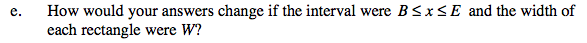x0 = B→height of rectangle = f(B)
x1 = B + width →height of rectangle = f(B + width)
xlast = E →height of rectangle = f(E)
xOneBeforeLast = E−width →height of rectangle = f(E − width)